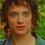# Manipulating Infinite Sums

!THE FOLLOWING MATH IS HIGHLY ILLOGICAL! Hey guys, I've been talking to my friends Josh Speckman and Nate Ji

So, you've probably seen the $1+2+3+4+5 \cdots = - \dfrac{1}{12}$. If not, here: We will define three sums: $S _1 = 1-1+1-1+1-1 \cdots$, $S _2 = 1-2+3-4+5-6 \cdots$, and $S _3 = 1+2+3+4+5 \cdots$.

Looking at the first sum, we see that $1-S _1=1-(1-1+1-1+1 \cdots) = 1-1+1-1+1-1 \cdots$. Thus, $S _1= \dfrac{1}{2}$.

Now we must solve the second sum. We get $S _2 +S _2 = 2S _2 = 1 -2+3-4+5 \cdots + 1 - 2 + 3 - 4 + 5 \cdots = 1 + (- 2 + 1) + (3-2) + (-4+3) \cdots = 1-1+1-1+1-1 \cdots = \dfrac{1}{2}$. Thus $2S _2 = \dfrac{1}{2} \Rightarrow S _2 = \dfrac{1}{4}$.

Now the last sum. We see that $S _3 - S _2 = 1+2+3+4+5 \cdots - (1 - 2 + 3 - 4 + 5 - 6 \cdots) = (1-1) + (2+2) +(3-3) + (4+4) \cdots = 4 + 8 + 12 + 16 \cdots = 4S _3$. So $S_3 - \dfrac{1}{4} = 4S _3 \Rightarrow 3S _3 = - \dfrac{1}{4} \Rightarrow S _3 = - \dfrac{1}{12}$.

Now, I was showing my friends this, and they decided to continue along the theme of not-quite valid mathematics. So, here it is:

$1+2+3+4+5\cdots$ is the sum of the first $\infty$ positive integers, so it is $\dfrac{\infty(\infty + 1)}{2} = \dfrac{\infty^2 + \infty}{2}$. Since this is equal to $-\dfrac{1}{12}$, we get $\dfrac{\infty^2+\infty}{2} = - \dfrac{1}{12}$,

so $\infty^2+\infty=-\dfrac{1}{6} \Rightarrow \infty^2+\infty+\dfrac{1}{6} = 0$,

and $\infty = \dfrac{-1 \pm \sqrt{\dfrac{1}{3}}}{2} \Rightarrow \infty =\boxed{ \dfrac{-3 \pm \sqrt{3}}{6}}$.

Yay infinite divergent series!Note by Frodo Baggins
7 years, 1 month ago

This discussion board is a place to discuss our Daily Challenges and the math and science related to those challenges. Explanations are more than just a solution — they should explain the steps and thinking strategies that you used to obtain the solution. Comments should further the discussion of math and science.

When posting on Brilliant:

• Use the emojis to react to an explanation, whether you're congratulating a job well done , or just really confused .
• Ask specific questions about the challenge or the steps in somebody's explanation. Well-posed questions can add a lot to the discussion, but posting "I don't understand!" doesn't help anyone.
• Try to contribute something new to the discussion, whether it is an extension, generalization or other idea related to the challenge.
• Stay on topic — we're all here to learn more about math and science, not to hear about your favorite get-rich-quick scheme or current world events.

MarkdownAppears as
*italics* or _italics_ italics
**bold** or __bold__ bold
- bulleted- list
• bulleted
• list
1. numbered2. list
1. numbered
2. list
Note: you must add a full line of space before and after lists for them to show up correctly
paragraph 1paragraph 2

paragraph 1

paragraph 2

[example link](https://brilliant.org)example link
> This is a quote
This is a quote
    # I indented these lines
# 4 spaces, and now they show
# up as a code block.

print "hello world"
# I indented these lines
# 4 spaces, and now they show
# up as a code block.

print "hello world"
MathAppears as
Remember to wrap math in $$ ... $$ or $ ... $ to ensure proper formatting.
2 \times 3 $2 \times 3$
2^{34} $2^{34}$
a_{i-1} $a_{i-1}$
\frac{2}{3} $\frac{2}{3}$
\sqrt{2} $\sqrt{2}$
\sum_{i=1}^3 $\sum_{i=1}^3$
\sin \theta $\sin \theta$
\boxed{123} $\boxed{123}$

## Comments

Sort by:

Top Newest

I DON'T UNDERSTAND YOU.

- 7 years, 1 month ago

Log in to reply

Well, infinity squared is still infinity and there are different types of infinity so you are practically stating that the divergent sum (zeta of -1) is equal to infinity AND minus one twelfth, and both things can be true.

- 7 years, 1 month ago

Log in to reply

×

Problem Loading...

Note Loading...

Set Loading...